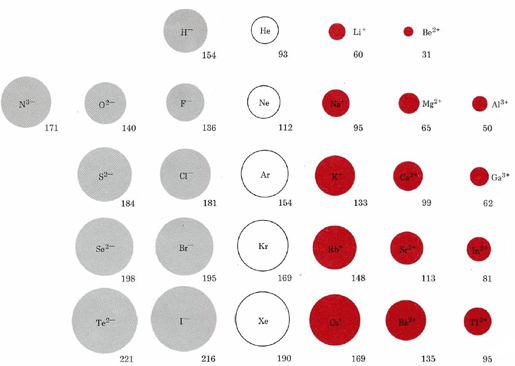6.19: Ionic Sizes

The size of an ion is governed not only by its electronic structure but also by its charge. This relationship is evident in the following figure comparing ionic radii. Ions in the first row of this figure, H, Li+, and Be2+, all have the same 1s2 electronic structure as the helium (He) atom, but differ in size due to the different number of protons each has in their nucleus.Species which have the same electronic structure but different charges are said to be isoelectronic. For any electronic series, such as H, He, Li+, Be2+, in which the nuclear charge increases by 1 each time, we find a progressive decrease in size due to the increasingly strong attraction of the nucleus for the electron cloud. Each row in the figure corresponds to an isoelectronic series involving a different noble-gas electron configuration.

As we move from the more negative to the more positive ions in each row, there is a steady decrease in size. If we move down any of the columns, ionic sizes increase due to the increasing principal quantum number of the outermost electrons. The sizes of singly charged cations, for example, increase in the following order: Li+ < Na+ < K+ < Rb+ < Cs+.

A further point of interest is the size of an ion relative to the atom from which it was formed. Recall the electron dot density diagram showing the formation of LiH. when the Li atom lost its electron and became a Li+ ion, its size decreased dramatically. Comparing atomic radii to the ionic radii above reveals that this is also true for the other alkali metals. For examples, check out the image below, which compares the atomic radii of the uncharged element to its ion. On the left are cations, which have lost an electron to become an ion and show decreases in size.Image Credit: By Popnose [CC BY-SA 3.0 (http://creativecommons.org/licenses/by-sa/3.0) or GFDL (http://www.gnu.org/copyleft/fdl.html)], via Wikimedia Commons

On the right side of the figure are the anions, which form when an element gains an electron. The added electron, as can been observed from the pattern above, increases the atomic radii. Since the added electron goes into a subshell which already has occupants, rather than starting on a new subshell, there is often very little change in size. This is clearly seen in the electron dot density diagram mentioned above, where formation of an H ion from an H atom produces no perceptible increase in size. Comparing the figure of atomic radii to ionic radii above, we also find that the van der Waals radii of nonmetals are only slightly smaller than the radii of their anions. However, some ions still experience fairly significant size changes, as can be seen in the figure above (anions are on the right side).

The sizes of the ions involved have considerable influence on both the chemical and physical properties of ionic compounds. There is a strong correlation, for example, between ionic size and the melting point of an ionic compound. Among the halides of sodium the melting point decreases in the order of NaF (995°C) > NaCl (808°C) > NaBr (750°C) > NaI (662°C). The larger the anion, the farther it is from the sodium ion, and the weaker the coulombic force of attraction between them. Hence the lower the melting point. When a very small cation combines with a very large anion, the resulting compound is less likely to exhibit the characteristic macroscopic properties of an ionic substance.